Geometry

# Triangles - Compare Similar Triangles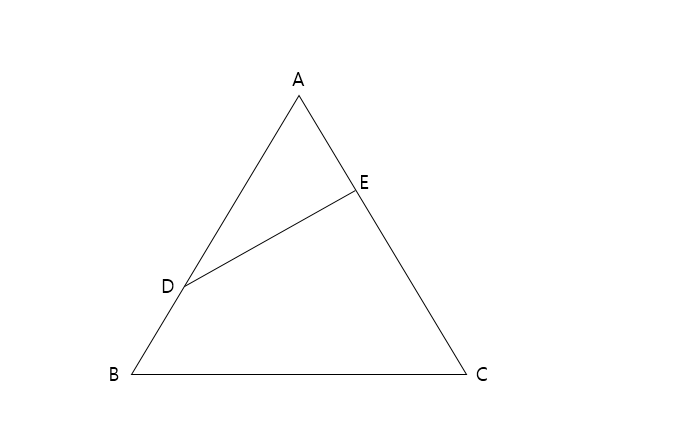In the above triangle, point D divides $\overline{AB}$ in the ratio $\ 2:1$ and point E divides $\overline{AC}$ in the ratio $\ 1:2.$ If the area of $\triangle ABC$ is $23,$ what is the area of $\triangle ADE?$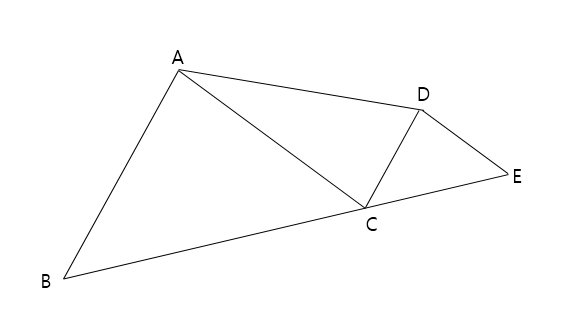Triangle $\triangle ABC$ is similar to $\triangle DCE$ with $\overline{AB} \parallel \overline{CD}.$ If the area of $\triangle ABC$ is $36$ and the area of $\triangle DCE$ is $9,$ what is the area of $\triangle ACD?$

Note: The above diagram is not drawn to scale.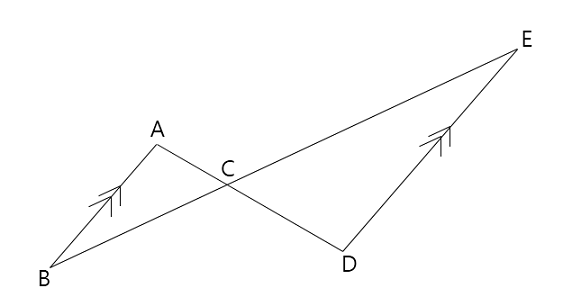$\overline{AB}$ is parallel to $\overline{DE}$, the length of $\overline{AC}$ is $3,$ and the length of $\overline{CD}$ is $11.$ If the area of $\triangle ABC$ is $4,$ what is the area of $\triangle CDE$ ?

Note: The above diagram is not drawn to scale.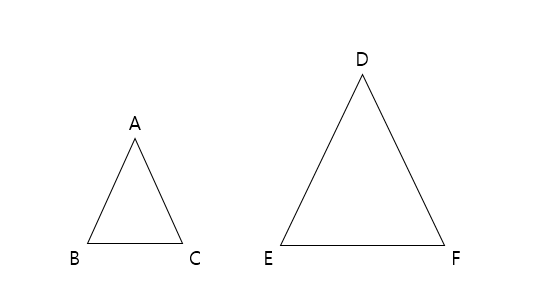Triangle $\triangle ABC$ is similar to $\triangle DEF$, and the ratio of their areas is $9:25.$ If the length of $\overline{DE}$ is $70,$ what is the length of $\overline{AB}$?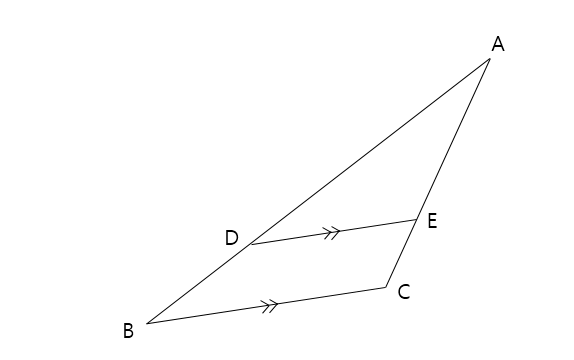$\overline{BC}$ is parallel to $\overline{DE}$, the length of $\overline{AD}$ is $7,$ and the length of $\overline{BD}$ is $3.$ If the area of $\triangle ABC$ is $17,$ what is the area of $\triangle ADE$ ?

Note: The above diagram is not drawn to scale.

×